# 配电网合环操作中的循环电流计算方法与降低措施研究Research on Calculation Method and Reduction Measures of Circulating Current in Closing Loop Operation in Distribution Network

DOI: 10.12677/TDET.2018.72007, PDF, HTML, XML, 下载: 813  浏览: 2,580

Abstract: In order to reduce the risk of distribution network operation, this paper calculates the power flow of distribution network based on the equivalent circuit diagram of distribution network, studies the calculation method of circulating current caused by loop closing operation of distribution network is studied, and puts forward a load control strategy to reduce circulating current, then uses the MATLAB/Simulink simulation software to build a simulation model of the operation of the distribution network, and the proposed control strategy to reduce the circulating current is simulated. The results verify the effectiveness of the proposed method.

1. 引言

2. 循环电流实时计算方法研究

$\Delta {U}_{a}={U}_{a1}-{U}_{a0}$ (1)

$\Delta {U}_{b}={U}_{b1}-{U}_{b0}$ (2)

$Z=\frac{\Delta {U}_{a}-\Delta {U}_{b}}{I}$ (3)

${I}_{循环}=\frac{{U}_{a0}-{U}_{b0}}{Z}$ (4)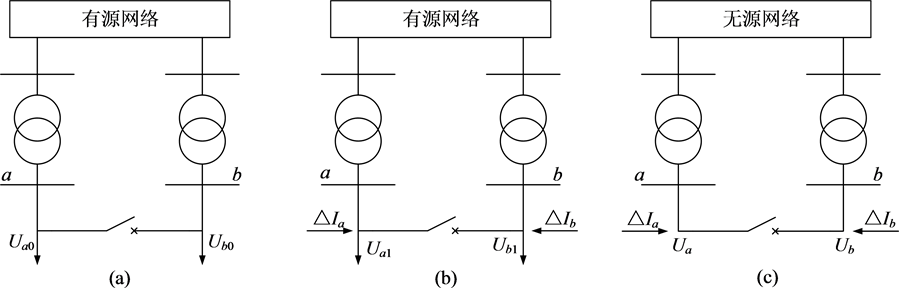Figure 1. Equivalent circuit diagram of combined loop current calculation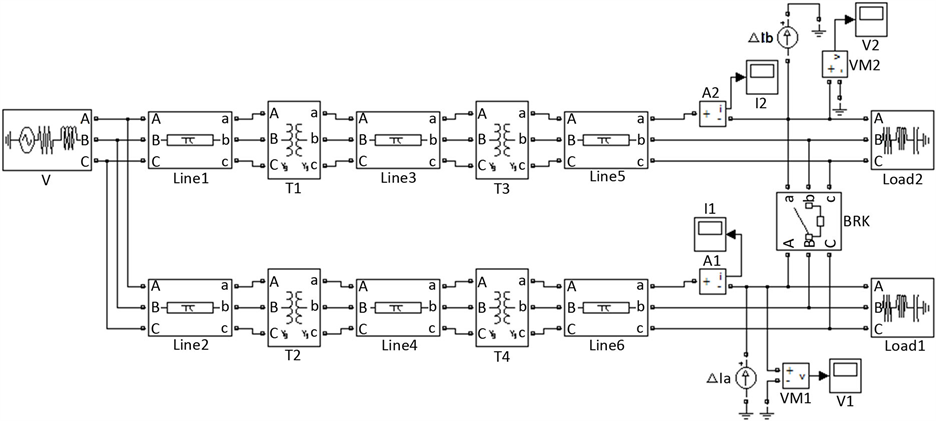Figure 2. Simulation circuit diagram of circulating current calculation

3. 合环操作影响判据

4. 提高合环转供电成功率的应对措施

4.1. 负荷控制Table 1. Current phasor measurement valuesTable 2. Voltage phasor measurement valuesTable 3. Calculated values of circulating currents

${U}_{AH2}={U}_{AL2}+\Delta {U}_{T2}={U}_{AL2}+{Z}_{T1}\frac{{S}_{AL2}}{U{}_{AL2}}$ (5)

${U}_{BH2}={U}_{BL2}+\Delta {U}_{T2}={U}_{BL2}+{Z}_{T2}\frac{{S}_{BL2}}{U{}_{BL2}}$ (6)

$\Delta {U}_{H2}=\frac{{S}_{AL2}{Z}_{T1}-{S}_{BL2}{Z}_{T2}}{U{}_{L2}}$ (7)

$S={S}_{AL2}+{S}_{BL2}$ (8)

$\Delta S={S}_{AL2}-{S}_{A}-{S}_{C}$ (9)

$\Delta S={S}_{BL2}-{S}_{B}-{S}_{D}$ (10)

${I}_{\text{c}}=\frac{\Delta S}{{U}_{L2}}$ (11)

${I}_{c}=\frac{\left({S}_{A}+{S}_{C}\right){Z}_{T1}-\left({S}_{B}+{S}_{D}\right){Z}_{T2}-\Delta {U}_{H2}{U}_{L2}}{\left({Z}_{T1}+{Z}_{T2}\right){U}_{L2}}$ (12)Figure 3. System closed-loop flow analysis diagram

4.2. 负变压器分接头调整

U1和U2为合环操作前合环开关两侧电压，对U1所属线路上的110 kV/10.5 kV变压器进行分接头调整，即U1可在其相量延长线上变化，U2保持不变。在对U1进行电压幅值调整时，影响合环电流大小的因素是电压相量差ΔU，因此应该以U2在U1相量上的投影U1t为准进行调整，此时合环点两侧电压差拥有最小值ΔUmin，分接头调整公式如下所示。

$k=\frac{{U}_{2}\mathrm{cos}\left({\theta }_{1}-{\theta }_{2}\right)-{U}_{1}}{{U}_{1}}$ (13)

5. 仿真与分析

5.1. 负荷控制仿真

5.1.1. 负荷1有功功率控制

5.1.2. 其他负荷功率控制Figure 4. Voltage phasor diagram for transformer tap adjustmentFigure 5. Load control simulation simplified diagram

5.1.3. 仿真步长为1 MW下的功率控制Table 5. Load 1 circulating current in active power control process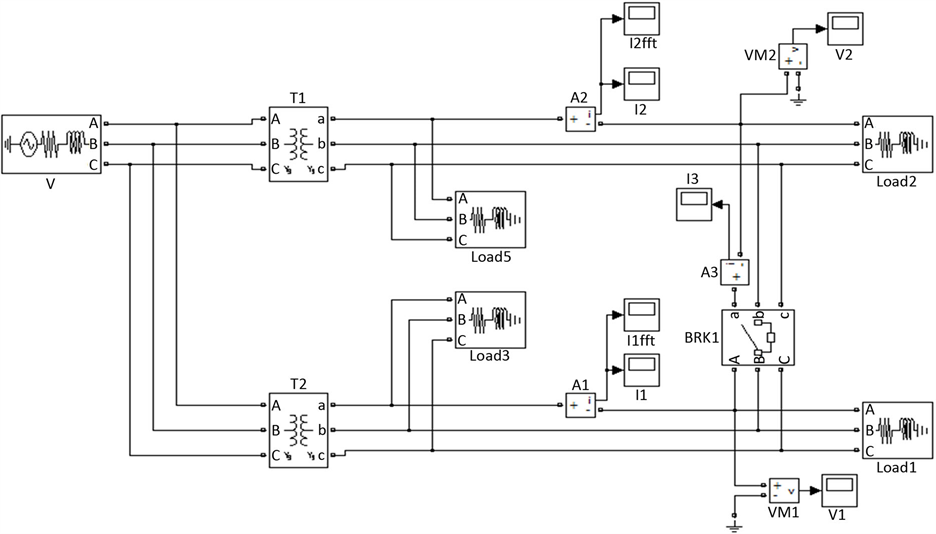Figure 6. Load control simulation circuit diagram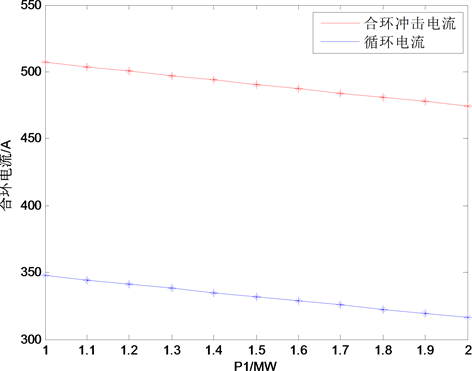Figure 7. Load 1 active power control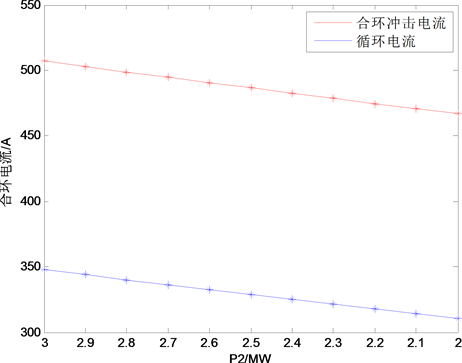(a)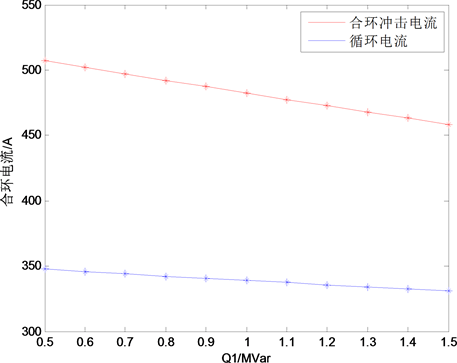(b)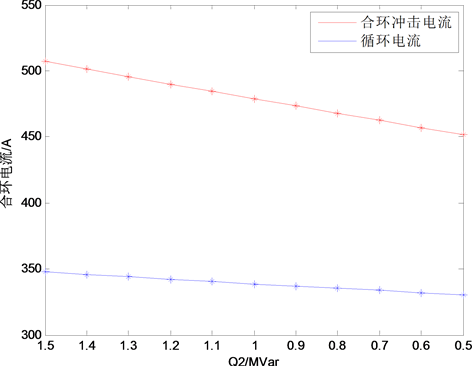(c)

Figure 8. Other load power control; (a) Load 1 reactive power control; (b) Load 2 active power control; (c) Load 2 reactive power control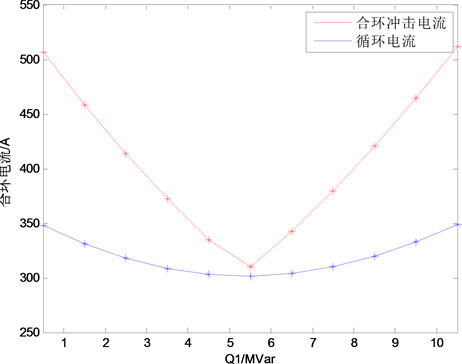(a)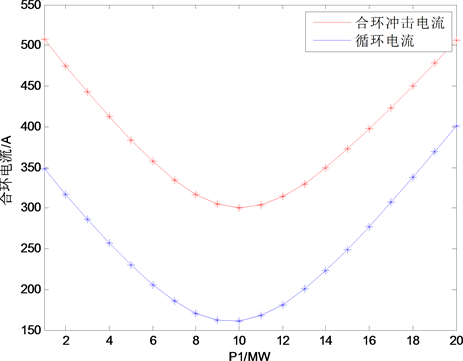(b)

Figure 9. Load 1 power control (a) Load 1 active power control; (b) Load 1 reactive power control

5.2. 变压器分接头调整仿真

6. 结论

1) 本文所提基于叠加原理的电流注入法测量系统等效阻抗，进而计算合环操作引起的循环电流具有实时性和直观性的优点。

2) 通过采用提高合环点两侧负荷对称性的负荷控制策略，特别是以减负荷达到提高对称性的方式，能有效降低合环操作引起的循环电流大小，进而降低合环操作的风险，提高作业过程中系统运行的安全Table 6. Transformer tap changer parametersTable 7. Loop current in the tapping processFigure 10. Load 1 active power control

3) 通过合理调整变压器分接头，控制合环开关两侧电压幅值差，能有效减小合环电流，提高合环成功率。

4) 本文对合环操作中降低循环电流的研究中提出了合理的负荷控制策略，实际工程中可以通过投切电容器或电抗器等措施实现，今后应对这些具体的负荷控制措施的实施过程及其对系统安全稳定的影响做深入研究。另外在变压器分接头调整的研究中可知合环点两侧电压相角差也是影响循环电流的重要因素之一，目前国内没有较好的解决办法，因此今后应该重点研究降低合环点两侧电压相角差的有效措施。

  叶凌. 利用综合不停电作业法进行负荷转供的研究[C]//决策论坛——政用产学研一体化协同发展学术研讨会论文集(下). 2015.  潘浩辉. 惠州10 kV配网合环转电对继电保护的影响研究[D]. 华北电力大学(北京), 2011.  刘健, 孙泉, 张小庆, 等. 配电网合环分析与合环条件判断[J]. 电力系统自动化, 2014, 38(11): 130-135.  Cheng, T. and Cheng, L.S. (2001) Study on Electromagnetic Loop Network Operation of Power Systems. Central China Electric Power.  席云华. 广州地区10 kV电网合环供电理论研究[J]. 科学技术创新, 2013(26): 18-20.  Zou, J.X., Zhou, G.B., Ke, F.U., et al. (2010) Electro-magnetic Loop Closing Calculation Model and Experimental Analysis on 10 kV Distribution Network. Power System Protection & Control.  刘洋. 10 kV配网合环电流计算方法研究[J]. 供用电, 2016, 33(4): 54-58.  谭明甜. 低压配电网环网系统设计、实现及可靠性分析[D]. 华北电力大学(北京), 2016.  郭添根. 浅析城市配电网存在问题及规划改造思路[J]. 军民两用技术与产品, 2014(17).  李旭前, 石连生, 吕泽阳, 等. 10 kV配电网合环倒负荷研究[J]. 云南电力技术, 2014, 42(2): 41-44.  赵国庆, 朱华锋. 关与10 kV城市配电网建设与电气设计[J]. 建材发展导向, 2010(2S): 105-106.  邬溢生. 10 kV配电网络合环转供电操作的可行性分析及实际应用[J]. 中国新技术新产品, 2013(20): 159-160.  王海燕, 曾江, 刘刚. 国外配网自动化建设模式对我国配网建设的启示[J]. 电力系统保护与控制, 2009, 37(11): 125-129.  杨鹏伟. 配电网合环分析计算与应用研究[D]. 华北电力大学(北京), 2016.  Thongkrajay, U. and Kulworawanichpong, T. (2008) Convergence Improvement of Gauss-Seidel Power Flow Solution Using Load Transfer Technique. Iasted International Conference on Model-ling, Identification and Control, ACTA Press, 1-6.